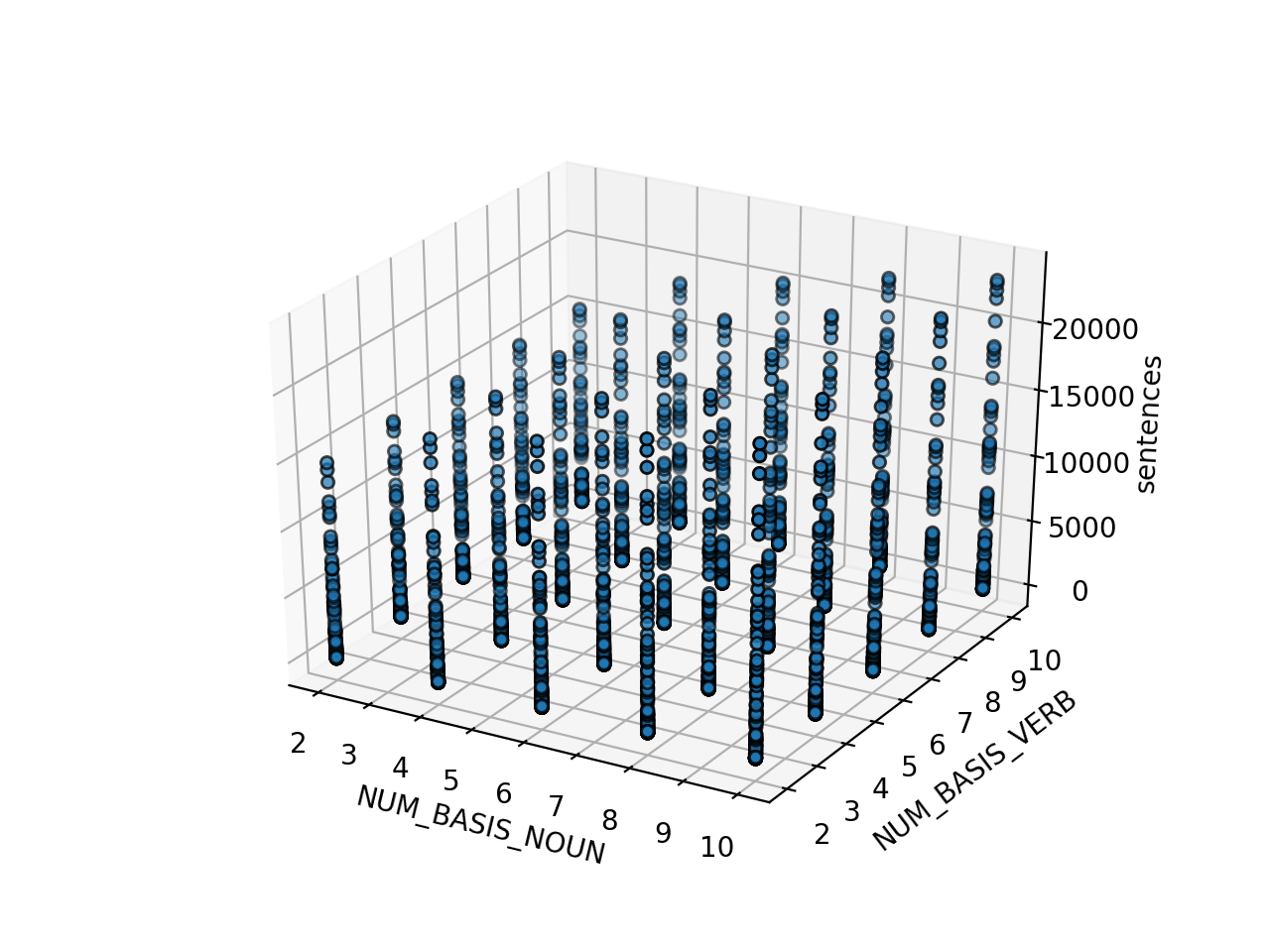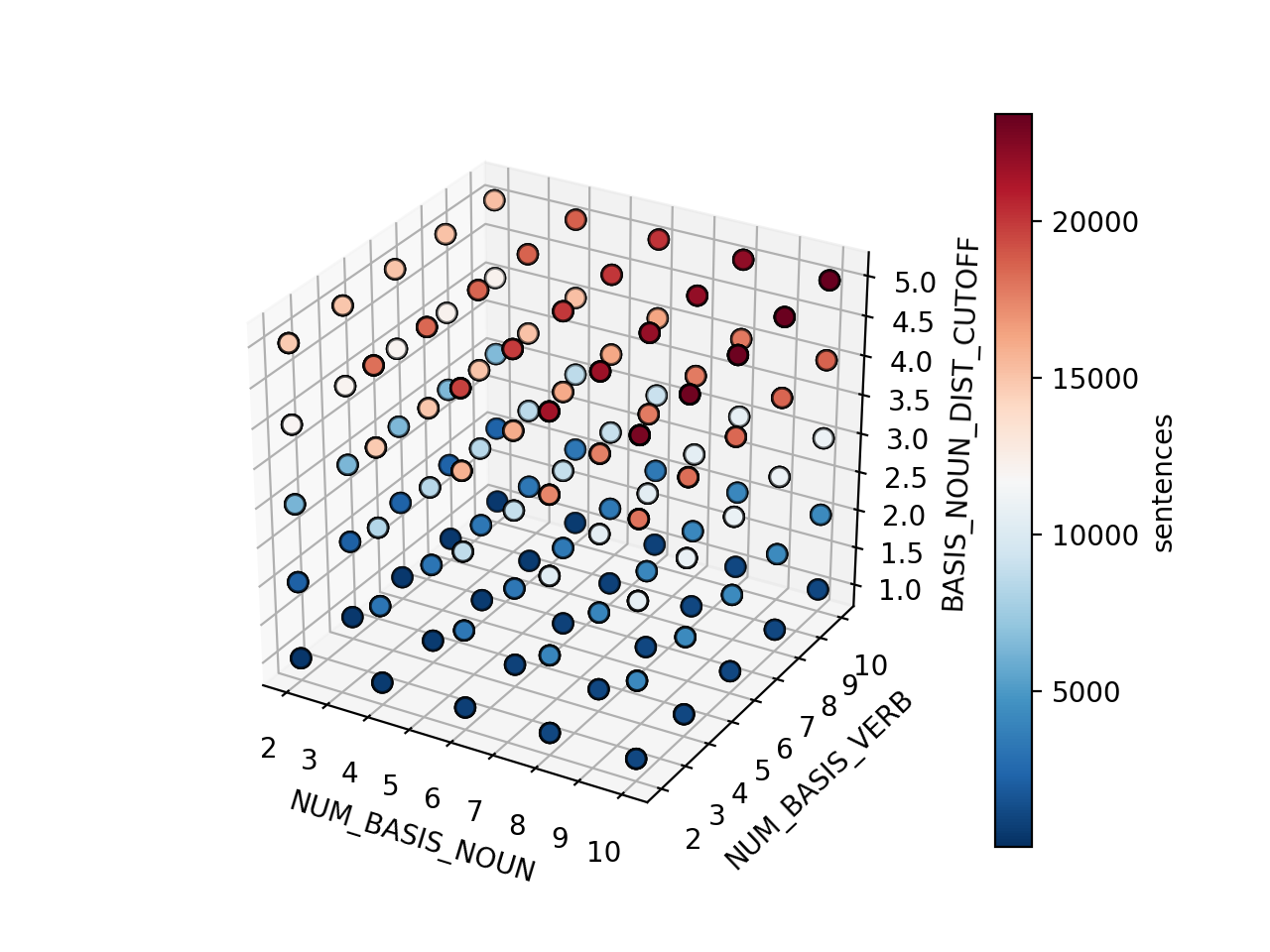# QNLP

Quantum Natural Language Processing

# Effect of pre-processing parameters on encoding patterns

%matplotlib notebook
import matplotlib.pyplot as plt
from mpl_toolkits.mplot3d import Axes3D
import pandas as pd

from matplotlib.ticker import MaxNLocator


Pre-processing our data is a necessary step to determine how one maps language tokens to quantum states for encoding. We can choose a variety of methods to associate tokens with one another with variable degrees of control. While it is possible to fully saturate and encode data using the entirely available pattern-set, fine tuning of the problem space during pre-processing can be beneficial.

Here we explore the differences observed in available sentences, and subsequently unique encoding patterns by controlling the following set of variables:

• Number of basis elements for noun and verb sets (NUM_BASIS_NOUN and NUM_BASIS_VERB)
• Basis-to-composite token cutoff distance for association (BASIS_NOUN_DIST_CUTOFF and BASIS_VERB_DIST_CUTOFF)
• Composite verb to composite noun cutoff distance for association (VERB_NOUN_DIST_CUTOFF)

As measurable outputs we can observe the number of sentences of composite tokens the preprocessing steps create, as well as the number of unique patterns, based upon tensoring the composite tokens basis element sets.

While encoding a large number of patterns may be possible, the upper limit of which is determined by the number of encoding patterns available for the chosen schema (number of noun patterns^2 * number of verb patterns), often it is more intructive to examine a sparsely occupied set of state.

Assuming the pre-processing stage has successfully chosen the token order mapping for the given sets of basis elements, few encoded tokens relative to the total available token-space will allow the same observation of the method for comparison. As such, we opt to choose parameters that follow this approach. It should also more easily allow comparisons with data that has not been encoded, where meaning is still preserved due to the methods proposed to set up the procedure.

"""
Here we give a file, with each line being a dictionary of the parameters

NUM_BASIS_NOUN, NUM_BASIS_VERB, BASIS_NOUN_DIST_CUTOFF, BASIS_VERB_DIST_CUTOFF, VERB_NOUN_DIST_CUTOFF

where each parameter above is a key with associated value of the variable run with.

sentences, patterns

hold the number of observed values for both quantities from the run.
"""
dict_file = "path_to_file.out"


We begin by loading the data file line by line and encoding it into a pandas dataframe object. We do this to easily perform filtering and selection of the data .

df = pd.DataFrame({})
with open(dict_file) as file:
for l in file:
dict_line = eval(l)
dict_line = {k:[v] for k,v in dict_line.items()}
df_tmp = pd.DataFrame(dict_line)
df = df.append(df_tmp)

df = df.reset_index(drop=True)
df


sentences patterns NUM_BASIS_NOUN NUM_BASIS_VERB BASIS_NOUN_DIST_CUTOFF BASIS_VERB_DIST_CUTOFF VERB_NOUN_DIST_CUTOFF
0 3 3 2 2 1 1 1
1 14 7 2 2 1 1 2
2 54 8 2 2 1 1 3
3 76 8 2 2 1 1 4
4 111 8 2 2 1 1 5
... ... ... ... ... ... ... ...
3120 116 384 10 10 5 5 1
3121 1872 967 10 10 5 5 2
3122 7534 1000 10 10 5 5 3
3123 14149 1000 10 10 5 5 4
3124 23424 1000 10 10 5 5 5

3125 rows Ã— 7 columns

With the data loaded, we may now slice and view it in any way to observe the relationships between the parameters and recorded sentences and patterns. Given we have a 5D parameter space, we can choose to observe flattened data over the non-observed, as below:

fig = plt.figure()
plot_order = ["NUM_BASIS_NOUN", "NUM_BASIS_VERB", "sentences"]
p3d = ax.scatter3D(
df[plot_order],
df[plot_order],
df[plot_order],
cmap="RdBu_r",
edgecolors='k'
)
ax.set_xlabel(plot_order)
ax.set_ylabel(plot_order)
ax.set_zlabel(plot_order)

<IPython.core.display.Javascript object>Text(0.5, 0, 'sentences')


or be more clever, and encode additional information into the size and colour of the scatter plot, as so:

fig = plt.figure()
plot_order = ["NUM_BASIS_NOUN", "NUM_BASIS_VERB", "BASIS_NOUN_DIST_CUTOFF", "sentences"]
p3d = ax.scatter3D(
df[plot_order],
df[plot_order],
df[plot_order],
s=df["BASIS_VERB_DIST_CUTOFF"]*10,
c=df[plot_order],
cmap="RdBu_r",
edgecolors='k'
)
ax.set_xlabel(plot_order)
ax.set_ylabel(plot_order)
ax.set_zlabel(plot_order)
cb = plt.colorbar(p3d)
cb.set_label(plot_order[-1], rotation=90)

<IPython.core.display.Javascript object>As above sentences, we can mirror the procedure for unique patterns as:

fig = plt.figure()
plot_order = ["NUM_BASIS_NOUN", "NUM_BASIS_VERB", "BASIS_NOUN_DIST_CUTOFF", "VERB_NOUN_DIST_CUTOFF", "patterns"]
p3d = ax.scatter3D(
df[plot_order],
df[plot_order],
df[plot_order],
s=df[plot_order]*10,
c=df[plot_order],
cmap="RdBu_r",
edgecolors='k'
)
ax.set_xlabel(plot_order)
ax.set_ylabel(plot_order)
ax.set_zlabel(plot_order)
cb = plt.colorbar(p3d)
cb.set_label(plot_order[-1], rotation=90)

<IPython.core.display.Javascript object>